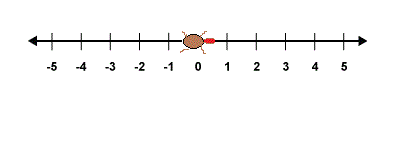# Hop on over

Logic Level 3Brilli the bug is constrained to move on a number line ranging $[-\infty ,\infty ]$. It starts at 0 and can go either left or right but moves in a way such that the $i$th 's move it takes $i$ steps.

What is the minimum number of moves required to reach 32 on the number line.

Details and Assumptions:

• As an explicit example to reach position $3$ it takes a minimum of $2$ moves $0\rightarrow 1 \rightarrow 3$

• The $i$-th move can be done with as a combination of left and right movement

×